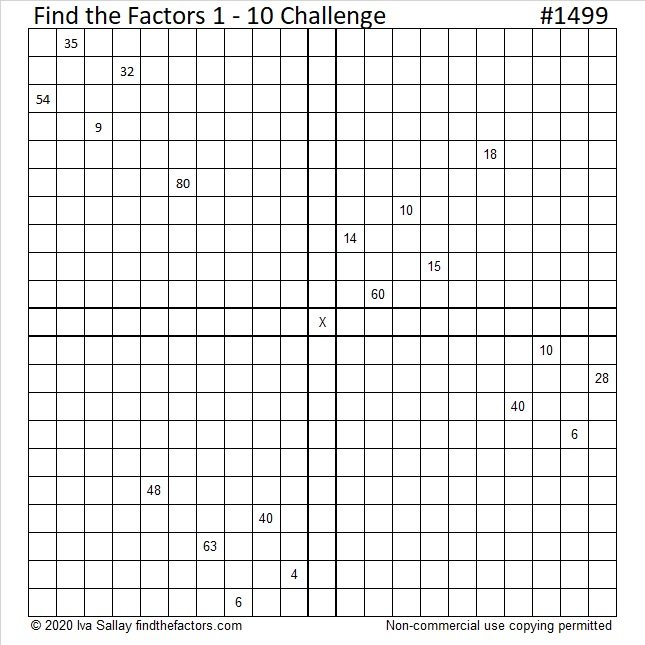# 1499 Challenge Puzzle

### Today’s Puzzle:

Use the 19 clues, logic, and the multiplication facts from a 10 × 10 multiplication table to find the unique solution of this Find the Factors Challenge puzzle. Good luck!### Factors of 1499:

• 1499 is a prime number.
• Prime factorization: 1499 is prime.
• 1499 has no exponents greater than 1 in its prime factorization, so √1499 cannot be simplified.
• The exponent in the prime factorization is 1. Adding one to that exponent we get (1 + 1) = 2. Therefore 1499 has exactly 2 factors.
• The factors of 1499 are outlined with their factor pair partners in the graphic below.How do we know that 1499 is a prime number? If 1499 were not a prime number, then it would be divisible by at least one prime number less than or equal to √1499. Since 1499 cannot be divided evenly by 2, 3, 5, 7, 11, 13, 17, 19, 23, 29, 31, or 37, we know that 1499 is a prime number.

### More Facts about the Number 1499:

1499 is the difference of two consecutive squares:
750² – 749² = 1499

oeis.org reminds us that 149, 199, and 499 are also prime numbers, so taking away one digit from 1499 always leaves a prime number.